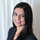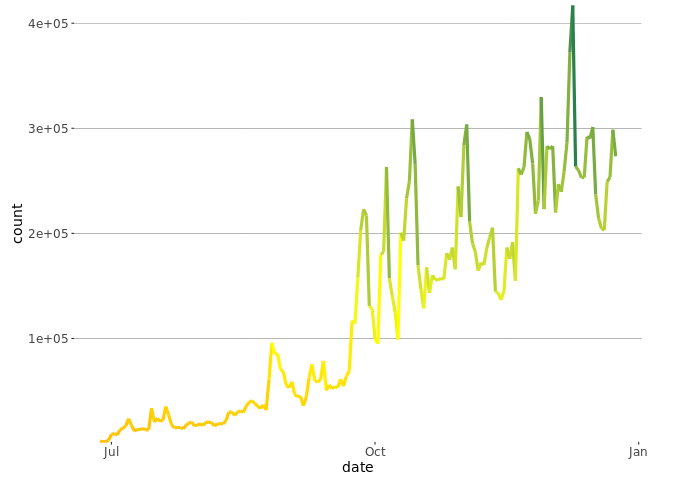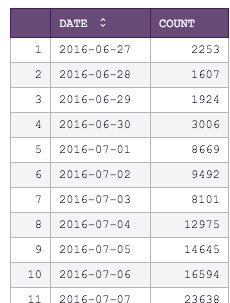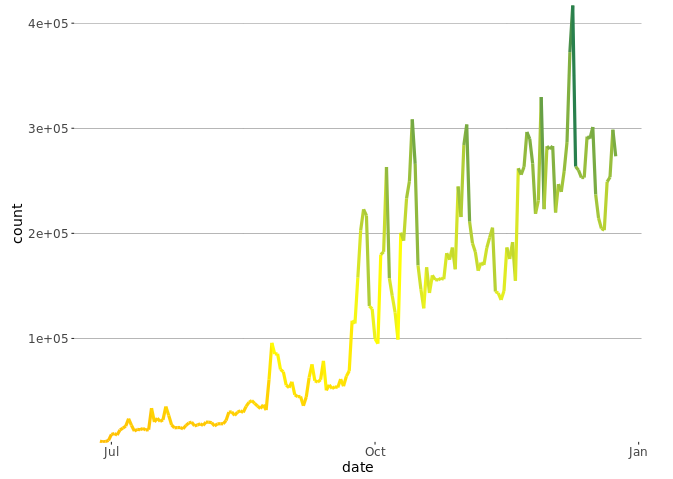# Plotting a Gradient Line in RColor is an extremely useful visualization tool. On a line chart, a color gradient can be used to emphasize the highs and lows. Using the Python / R integration in Periscope, we can definitely achieve this look.

Here's the SQL output for the number of gameplays for a fictional company.From here, we apply the following R code to create a line chart where lower values are marked in red and higher values are marked in green.

```# SQL output is imported as a dataframe variable called "df"
# Use Periscope to visualize a dataframe or show text by passing data to periscope.table() or periscope.text() respectively. Show an image by calling periscope.image() after your plot.
library(ggplot2)

output <- ggplot(df, aes(x = date, y = count, color = count )) +
geom_line(size = 1.5)  +
scale_colour_gradient2(low = "red", mid = "yellow" , high = "seagreen", midpoint=median(df\$count)) +
theme(legend.position="none")

#make it look like other Periscope charts
output <- output + scale_y_continuous(expand = c(0, 0))
output <- output +
theme(panel.background = element_rect(fill = "transparent"),panel.grid.major.x = element_blank(),panel.grid.major.y =element_line(size=.15, color="gray15"),axis.text=element_text(family='NimbusSan',size=12),axis.title.x=element_text(family='NimbusSan',size=14),axis.title.y=element_text(family='NimbusSan',size=14))
output <- output + theme( rect = element_rect(fill = "transparent"))

periscope.image(output)```

This generates the below output. Pretty neat, right?